Next: Helium Atom Up: Variational Methods Previous: Introduction

Variational Principle

Suppose that we wish to solve the time-independent Schrödinger equation(1167)

whereis a known (presumably complicated) time-independent Hamiltonian. Let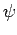be a normalized trial solution to the above equation. The variational principle states, quite simply, that the ground-state energy,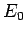, is always less than or equal to the expectation value ofcalculated with the trial wavefunction: i.e.,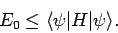(1168)

Thus, by varyinguntil the expectation value ofis minimized, we can obtain an approximation to the wavefunction and energy of the ground-state.

Let us prove the variational principle. Suppose that the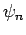and theare the true eigenstates and eigenvalues of: i.e.,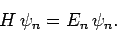(1169)

Furthermore, let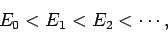(1170)

so that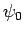is the ground-state,the first excited state, etc. Theare assumed to be orthonormal: i.e.,(1171)

If our trial wavefunctionis properly normalized then we can write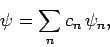(1172)

where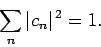(1173)

Now, the expectation value of, calculated with, takes the form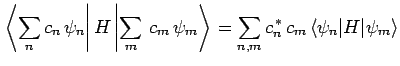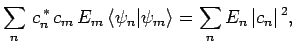(1174)

where use has been made of Eqs. (1169) and (1171). So, we can write(1175)

However, Eq. (1173) can be rearranged to give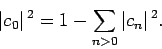(1176)

Combining the previous two equations, we obtain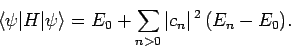(1177)

Now, the second term on the right-hand side of the above expression is positive definite, sincefor all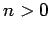[see (1170)]. Hence, we obtain the desired result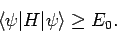(1178)

Suppose that we have found a good approximation,, to the ground-state wavefunction. Ifis a normalized trial wavefunction which is orthogonal to(i.e.,) then, by repeating the above analysis, we can easily demonstrate that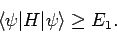(1179)

Thus, by varyinguntil the expectation value ofis minimized, we can obtain an approximation to the wavefunction and energy of the first excited state. Obviously, we can continue this process until we have approximations to all of the stationary eigenstates. Note, however, that the errors are clearly cumulative in this method, so that any approximations to highly excited states are unlikely to be very accurate. For this reason, the variational method is generally only used to calculate the ground-state and first few excited states of complicated quantum systems.Next: Helium Atom Up: Variational Methods Previous: Introduction
Richard Fitzpatrick 2010-07-20